# 模型评价指标说明与实践–混淆矩阵的说明2019年7月4日07:06:17

3554字阅读11分50秒

## 指标说明

### TP, TN, FP, FN的简单说明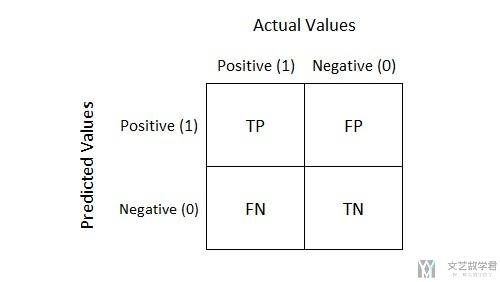• TP: 预测为1(Positive)，实际也为1(Truth-预测对了)
• TN: 预测为0(Negative)，实际也为0(Truth-预测对了)
• FP: 预测为1(Positive)，实际为0，也就是 Negative(False-预测错了)
• FN: 预测为0(Negative)，实际为1，也就是 Positive(False-预测错了)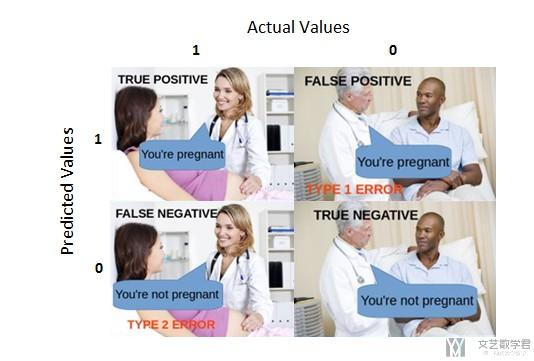True Positive:

• Interpretation: You predicted positive and it's true.
• You predicted that a woman is pregnant and she actually is.

True Negative:

• Interpretation: You predicted negative and it's true.
• You predicted that a man is not pregnant and he actually is not.

False Positive: (Type 1 Error)

• Interpretation: You predicted positive and it's false.
• You predicted that a man is pregnant but he actually is not.

False Negative: (Type 2 Error)

• Interpretation: You predicted negative and it's false.
• You predicted that a woman is not pregnant but she actually is.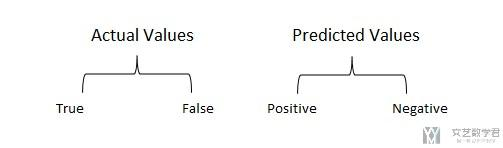### 一些衍生的指标--FPR, FNR, FAR

The false alarm rate (FAR) is the average ratio of themisclassified to classified records either normal or abnormal as denoted in Eq. (22)(下面的等式).

It is designed from Eqs. (20) and (21) to calculate the false positive rate (FPR) and the false negative rate (FNR), respectively.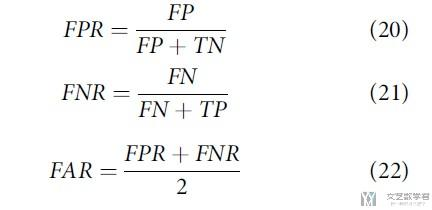### Accuracy/Precision/Recall的定义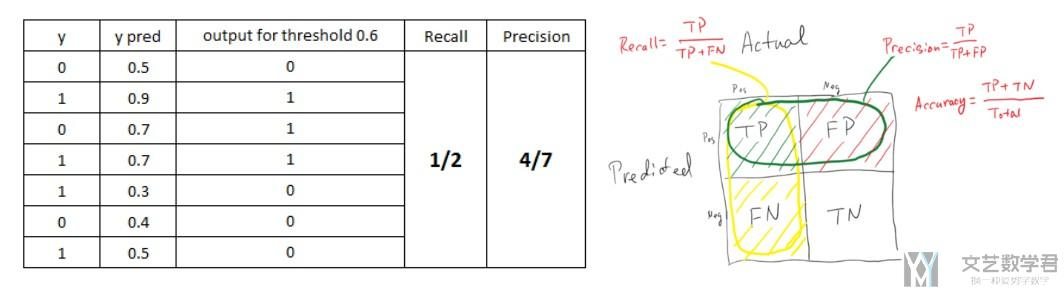• 对于上面图片的情况来说:
• TP=2
• FP=1
• FN=2
• TN=2
• Accuracy = (预测正确的样本数)/(总样本数)=(TP+TN)/(TP+TN+FP+FN)
• Precision = (预测为1且正确预测的样本数)/(所有预测为1的样本数) = TP/(TP+FP), 这个值也是越大越好, 上面例子的计算是2/3, 上面图片好像是错的。
• Recall = (预测为1且正确预测的样本数)/(所有真实情况为1的样本数) = TP/(TP+FN), 这个值是越大越好, 上面得例子中是2/4=0.5

#### F1值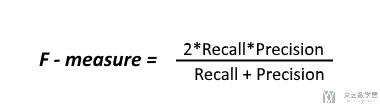• F1 = 2*(Precision*Recall)/(Precision+Recall)

### Micro Average vs Macro average

A macro-average will compute the metric independently for each class and then take the average (hence treating all classes equally).(macro-average相当于是对所有分类的指标进行平均)

A micro-average will aggregate the contributions of all classes to compute the average metric.(micro-average有点类似与加权平均, 他会考虑每个类的样本的个数, 在面对每一类数据量不平衡的时候会起到作用)

• Class A : 1 TP and 1 FP
• Class B : 10 TP and 90 FP
• Class C : 1 TP and 1 FP
• Class D : 1 TP and 1 FP

Class A, Class C, Class D的Precision是一样的, 都是0.5.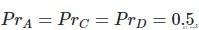Class B的Precision是0.1.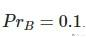• A macro-average will then compute(就是每一类的Pr求平均):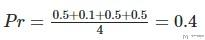• A micro-average will compute(这个会根据样本的个数重新进行计算):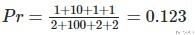## 简单的实践

sklearn说明文档 : sklearn.metrics.classification_report

1. from tools import *
2. # 模型的评估
3. display_model_performance_metrics(true_labels=testLabel['Attack_types'].to_numpy(),
4.                              predicted_labels=testLabel['predictions'].to_numpy(),
5.                                  classes=[0,1,2,3,4])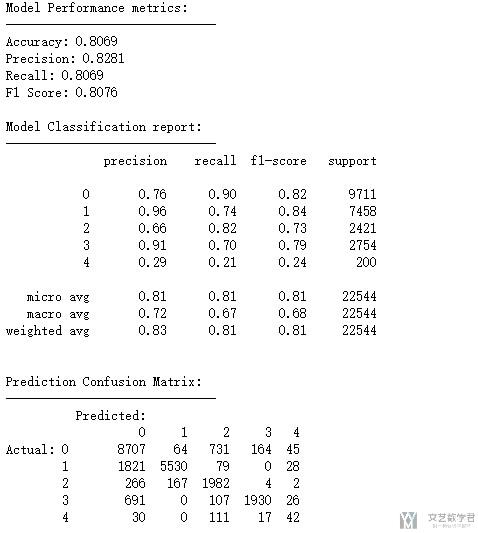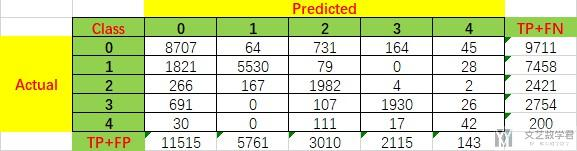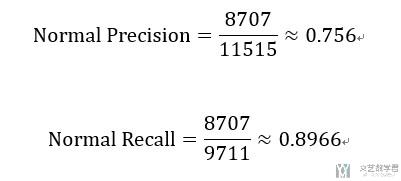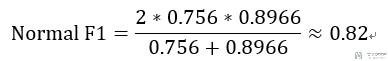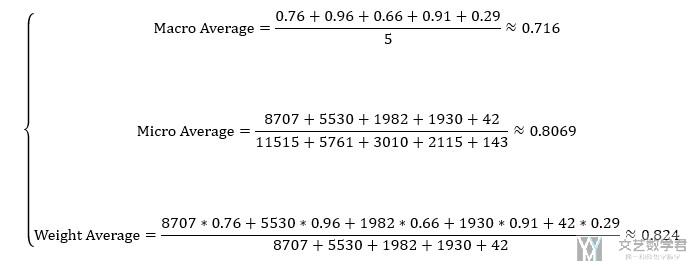• 微信公众号
• 关注微信公众号
•• QQ群
• 我们的QQ群号
•• 本文由 发表于 2019年7月4日07:06:17
• 转载请务必保留本文链接：https://mathpretty.com/10722.html